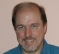Started by 6 years ago7 replieslatest reply 6 years ago407 views

Hello,

I have an application that plots a spectrum originating from a software defined radio.  I have read a number of articles of how to remove the spike at DC but I have not had any luck in doing so.

I have started with a basic approach: I collect 16384 samples each of the I and Q signals and average them individually and then subtract the average from each I and Q sample.  I see no change what so ever in the amplitude of the spike.  Am I doing something wrong here?

Alternatively, if would seem that correcting this on the spectrum itself, would be a lot easier.  As an example, I could provide the users with a calibration routine that would interactively allow them to reduce the amplitude of the spike.  In the software, the value obtained would be simply subtracted from the DC spike This routine would also need a width adjustment centered around the DC spike since the signal is spread a bit too.

Is this feasible? What is the shape of the peak in general?

Thanks for any insight.

Tom

[ - ]Since subtracting the mean does not work, it sounds like you have more low-frequency noise than just at dc.  How about a high-pass filter having zeros at dc and a pass-band that starts at the first interesting frequency?

[ - ]Look at the actual FFT bins.  If you see a zero at bin 0, surrounded by tall numbers, then (as JOS said) you've got a spike at more than DC.

Eliminating this after FFT-ing is problematic if the DC content is large -- if most of your energy is in just one bin, it'll reduce the available numerical accuracy for the other bins.  If the DC content doesn't stand out all that much, then "killing" it in the frequency domain may not be a bad idea.

[ - ]Thanks for the suggestions.

I have however determined that subtracting the mean does reduced the central spike a bit.  It lowers it from a height of 15dbm to 10dbm.  Should it not just eliminate it?

I guess one question is what is the average signal?  There are a lot of signals throughout the band of interest so this is not a true average of the baseline.

[ - ]If you use a rectangular window encompassing the entire length of the signal, then the dc component (bin 0) of the de-meaned signal's FFT will be exactly zero, to numerical precision.  If you have a different window, then bin 0 contains the sum of the dc component weighted by that window.

[ - ]I believe that dc component is either dc offset which will become zero if this offset is removed by subtracting mean or dc component is part of a flat topped spectrum in which case you don't want to make it zero and end up as a notch at dc bin.

Kaz

[ - ]Subtracting the mean from the signal should, indeed, reduce the DC content to zero.

So something is going on either with your FFT or your adjustment.  Keep in mind that the FFT is just a complicated (and fast) way of finding the Fourier series of a set of sampled-time data.  And, the frequency = 0 element of any Fourier series is a scaled version of the mean value of the signal.

If you are, indeed, reducing the mean of the raw data to zero and bin 0 of the FFT isn't zero, then something's wrong with your FFT.  If you're doing your operation and the sum of the series you're FFT-ing isn't zero, then you're doing something else wrong.

You're not subtracting the mean and then windowing, are you?  If you are, then that could affect the signal mean.

[ - ]